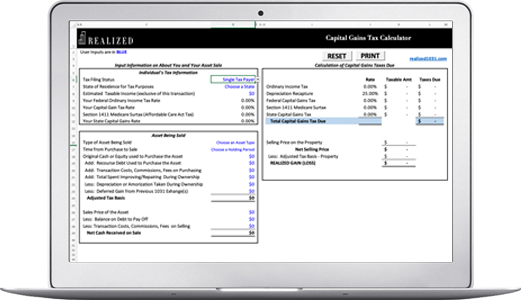Capital Gains Yield 2020-01-22 08:00:00

The capital gains yield of a stock represents the absolute return from time 1 to time 2. It is calculated using the formula: (p2 - p1) / p1, where p1 is the price at time 1 and p2 is the price at time 2. For example, the price of the stock ABC is \$100 on day 1. On day 5, it is \$105. Its capital gains yield is (105 - 100) / 100 = 0.0105 or a 1.05% gain.

Capital gains yield is often used to find out the return of a stock from the time of purchase to the time of sale. In the above example, the investor would have purchased the stock at \$100 and netted a \$5 gain in dollar terms, resulting in a 1.05% gain. Capital gains yield doesn’t include stock dividends, which is considered total return.